# A Tale of Two Flat‑Maps

Episode #10 • Mar 27, 2018 • Free Episode

Swift 4.1 deprecated and renamed a particular overload of `flatMap`. What made this `flatMap` different from the others? We’ll explore this and how understanding that difference helps us explore generalizations of the operation to other structures and derive new, useful code!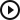A Tale of Two Flat‑Maps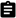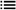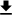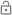This episode is free for everyone.

### Subscribe to Point-Free

Access all past and future episodes when you become a subscriber.

See plans and pricing

### Introduction

A change was proposed for Swift 4.1 that would deprecate an overload of `flatMap` that was a little different from the other `flatMap`s, and provide a new name for this method. The change was met with mixed reception, but ultimately was accepted, although the name was further changed due to community feedback.

We want to examine why this change was proposed in the first place, and why perhaps the first proposed name might have left us open to further concepts. We hope to show that sometimes naming really matters, and can help us leverage previous intuitions in new, unexpected ways.

### A tale of two flatMaps

Early on, Swift embraced functional programming patterns by providing functions like `map`, `filter`, `reduce`, and `flatMap` in the standard library.

Swift 1.0 shipped with `flatMap` defined on `Array` with the signature that we are all familiar with:

``````// extension Array {
//   func flatMap<B>(_ f: @escaping (Element) -> [B]) -> [B] {
//   }
// }
``````

This is incredibly useful for chaining operations together that return arrays.

For example, given a string holding a comma and newline separated list of values:

``````let csv = """
1,2,3,4
3,5,2
8,9,4
"""
``````

What if we wanted to process this string and extract all values between the commas?

We can start by splitting on newlines.

``````csv
.split(separator: "\n")
// ["1,2,3,4", "3,5,2", "8,9,4"]
``````

Now we want to dive into this array to further split each string on commas.

We’re familiar with using `map` to modify arrays.

``````csv
.split(separator: "\n")
.map { \$0.split(separator: ",") }
// [["1", "2", "3", "4"], ["3", "5", "2"], ["8", "9", "4"]]
``````

This returns nested arrays of values, but we want a flat array of values.

This is exactly what `flatMap` lets us do. It applies a function to each element of the array and then flattens it.

``````csv
.split(separator: "\n")
.flatMap { \$0.split(separator: ",") }
// ["1", "2", "3", "4", "3", "5", "2", "8", "9", "4"]
``````

Shortly thereafter, Swift introduced `flatMap` on `Optional` with the following signature:

``````// extension Optional {
//   func flatMap<B>(_ f: @escaping (Element) -> B?) -> B? {
//   }
// }
``````

This is useful for chaining operations together than return optionals.

For example, `String` has a failable initializer that takes data and returns an optional string:

``````String(data: Data(), encoding: .utf8)
// Optional("")
``````

As with arrays, we can `map` on optionals to transform the wrapped value. Say we want to transform our optional string into an integer.

``````String(data: Data(), encoding: .utf8)
.map(Int.init)
// Optional(nil)
``````

We got `nil` back because we were calling the `Int` initializer with an empty string, but something else is a little strange here. Is the type `Optional<Int>`?

``````_: Int? =  String(data: Data(), encoding: .utf8)
.map(Int.init)
// Value of optional type 'Int??' not unwrapped
``````

It’s not! Using `map` results in a double-optional `Int??`. We use `map` on an optional with an initializer that returned an optional, resulting in a nest of optionals! What we really wanted to use is `flatMap`.

``````_: Int? =  String(data: Data(), encoding: .utf8)
.flatMap(Int.init)
// nil
``````

This now compiles, which means our value is being flattened. It’s still returning `nil`, so let’s provide some data.

``````String(data: Data(), encoding: .utf8)
.flatMap(Int.init)
// Optional(7)
``````

With `flatMap` we were able to take our optional string, apply a function to its unwrapped value, and then take that optional result and flatten it back into a single optional integer.

There was a third `flatMap` on `Array` that blurred these worlds together: it took array elements and sent them through transformations that return optionals. Let’s look at an array of strings.

``````["1", "2", "buckle", "my", "shoe"]
``````

What if we wanted to transform each string into an integer? Using `map`, we get back the following:

``````["1", "2", "buckle", "my", "shoe"]
.map(Int.init)
// [{some 1}, {some 2}, nil, nil, nil]
``````

We’re given back an array where successful transformations are wrapped in an optional, while transformations that failed are `nil`.

If we used `flatMap` instead, we could discard those `nil`s and safely unwrap the integers.

``````["1", "2", "buckle", "my", "shoe"]
.flatMap(Int.init)
// [1, 2]
``````

This is incredibly useful! We do this kind of thing all the time. But this version of `flatMap` feels a bit different than the others since it’s mixing together some qualities of arrays with qualities of optionals.

Things become even more confusing when using both methods together. Let’s take our CSV example from earlier and further convert each value to an integer before adding them up.

``````csv.split(separator: "\n")
.flatMap { \$0.split(separator: ",") }
.flatMap { Int(\$0) }
.reduce(0, +)
// 41
``````

Here the first `flatMap` is in charge of getting all the values separated by commas and flattening them into a single array, and then the next `flatMap` tries to create an `Int` from the string, and discards any that fail to do so. These are two very different operations but we’re using the same name for them, making it difficult to tell them apart at a glance here.

We spend a lot of time thinking about types, particularly the shape of functions. Sometimes this can get lost in the definition of a method: the container type fades away from the declaration, and the function and argument names can make things a bit hazier. Let’s isolate these function signatures to the types themselves. We’ll use our free function syntax of `(Configuration) -> (Data) -> ReturnValue`:

``````// flatMap : ((A) -> [B]) -> ([A]) -> [B]
// flatMap : ((A) ->  B?) -> ( A?) ->  B?

// flatMap : ((A) ->  B?) -> ([A]) -> [B]
``````

One of these shapes is not like the others. The top two `flatMap`s operate with a single container type: `Array` and `Optional`, but the third `flatMap` operates on both containers at once.

If we desugar things, the generics look like this:

``````// flatMap : ((A) ->    Array<B>) -> (   Array<A>) ->    Array<B>
// flatMap : ((A) -> Optional<B>) -> (Optional<A>) -> Optional<B>

// flatMap : ((A) -> Optional<B>) -> (   Array<A>) ->    Array<B>
``````

What if we could think of the container `Array` and `Optional` types as generic types of their own? We can write things in such a way where we throw away their names to come up with something like this:

``````// flatMap : ((A) -> M<B>) -> (M<A>) -> M<B>
// flatMap : ((A) -> M<B>) -> (M<A>) -> M<B>

// flatMap : ((A) -> N<B>) -> (M<A>) -> M<B>
``````

Whoa, the first two signatures end up turning into the exact same thing! But with the third signature, we’re dealing with two different generic container types and the semantics become a lot more confusing. What exactly is `N` doing to produce an `M`? In the other versions, one can kind of make sense of the transform function having something to do with composing into the container type all over again. In this version, the `N` doesn’t really give us much to go on.

To make sense of it, we have to go back to concrete types.

``````// flatMap : ((A) -> B?) -> (M<A>) -> M<B>
``````

This is still a bit confusing. Can any `M` work with `Optional` in this way? Maybe this third `flatMap` isn’t as generic as the others.

### Optional promotion

There was another issue with overloading `flatMap` on `Array` for operations that return `Optional`s: optional promotion. For the sake of ergonomics, Swift automatically wraps values in the `Optional` type wherever an optional parameter requires. This can lead to code that’s much more succinct, and it can reduce some of the boilerplate burden on the engineer that would have otherwise needed to explicitly wrap values with `.some`, but type inference is a double-edged blade, and in the case of a closure that returns an optional result, anything is fair game.

Consider the following snippet:

``````[1, 2, 3]
.flatMap { \$0 + 1 }
// [2, 3, 4]
``````

This compiles, runs, and produces a result, but the semantics are strange: we’re not returning an optional from the provided closure. The compiler is automatically wrapping the value for us, like this:

``````[1, 2, 3]
.flatMap { .some(\$0 + 1) }
// [2, 3, 4]
``````

Wrapping our value manually shows that our logic here is a bit strange. We always return `.some` and never fail over to `nil`. Because this operation can never fail, we could have just used `map`.

``````[1, 2, 3]
.map { \$0 + 1 }
// [2, 3, 4]
``````

Because of optional promotion, any operation that works with `map` will also work with `flatMap`. It might even be tempting to avoid `map` in general because `flatMap` always seems to work, but `map` sometimes doesn’t. This is a bit unfortunate because we lose a bit of semantic meaning if we use `flatMap` everywhere. If we use both `flatMap` and `map`, we document operations that can and cannot fail explicitly.

Even worse, we can make changes to our types that we would expect to be compile time errors, but because of the overloaded `flatMap` and optional promotion it compiles fine but it has unexpected runtime behavior. For example, given a `User` struct with an optional name, and an array of users:

``````struct User {
let name: String?
}

let users = [User(name: "Blob"), User(name: "Math")]
``````

Given an array of users, we may be tempted to `map` over them and pluck out their names.

``````users
.map { \$0.name }
// [{some "Blob"}, {some "Math"}]
``````

But now we have this array of optional values. What we really wanted to use is `flatMap`.

``````users
.flatMap { \$0.name }
// ["Blob", "Math"]
``````

We might build a lot of code around a type like this, and one day we may change our `User` type to require a name. We’ve grown to appreciate that a powerful type system can help guide us through refactoring code whenever we change the way our type looks.

``````struct User {
let name: String
}
``````

When our code recompiles, what happens to our runtime behavior?

``````users
.flatMap { \$0.name }
// ["B", "l", "o", "b", "M", "a", "t", "h"]
``````

That’s unexpected! Maybe it’s a good thing that this outlier was renamed.

### compactMap and filterMap

So, given the complications that came up with the overloaded `flatMap` that was not quite like the other `flatMap`s, it was decided to deprecate it and introduce a new name. It is very important to remember that `flatMap` on arrays with transforms that return arrays, and `flatMap` on optionals that return optionals is not deprecated. It is only the one outlier method.

The name originally proposed was `filterMap`, in that you are mapping over the array and then discarding the `nil` values. It could be defined like so:

``````extension Array {
func filterMap<B>(_ transform: (Element) -> B?) -> [B] {
var result = [B]()
for x in self {
switch transform(x) {
case let .some(x):
result.append(x)
case let .none:
continue
}
}
return result
}
}
``````

After a bit of bike shedding on the evolution mailing list it was ultimately changed to `compactMap`, which is nice because it shares some prior art in Ruby, where `compact` is a method on arrays that discards `nil` values. Let’s go ahead and define `compactMap`:

``````extension Array {
func compactMap<B>(_ transform: (Element) -> B?) -> [B] {
var result = [B]()
for x in self {
switch transform(x) {
case let .some(x):
result.append(x)
case let .none:
continue
}
}
return result
}
}
``````

It’s just a rename, so we don’t have to change the body of the function.

### Generalizations of filterMap

One of the downsides of the `compactMap` name is that is has a strong ties to what we are doing with an array: we are “compacting” it to make it smaller by removing the `nil`s. This prevents us from seeing where `compactMap` might have broader applications.

But, one of the wonderful things about the `filterMap` name was that it could lead to some nice generalizations.

Let’s start by observing that a predicate `(A) -> Bool` on `A` naturally induces a function `(A) -> A?`:

``````func filterSome<A>(_ p: @escaping (A) -> Bool) -> (A) -> A? {
return { p(\$0) ? .some(\$0) : .none }
}
``````

It simply returns `.some` of the value when the predicate evaluates to `true`, and `.none` otherwise.

With this function we can now re-implement regularly ole `filter` on arrays:

``````func filter<A>(_ p: @escaping (A) -> Bool) -> ([A]) -> [A] {
return { \$0.filterMap(filterSome(p)) }
}
``````

Let’s try it out. We can create a filter for even integers.

``````filter { \$0 % 2 == 0 }
// ([Int]) -> [Int]
``````

And pipe an array through.

``````Array(0..<10)
|> filter { \$0 % 2 == 0 }
// [2, 4, 6, 8, 10]
``````

That’s pretty neat, but not immediately useful. We could already define `filter` ourselves or just use the one from the standard library. But, the reason we’d want to look at `filter` this way is that perhaps it will lead us to further generalizations.

One way to do this is to remember that `Either<A, B>` is a generalization of `Optional<A>`.

``````enum Either<A, B> {
case left(A)
case right(B)
}
``````

Instead of modeling the absence of an `A` with `nil`, we can supply a different value of type `B` in its place.

The function analogous to `filterSome` for `Either` would look something like this:

``````func partitionEither<A>(_ p: @escaping (A) -> Bool) -> (A) -> Either<A, A> {
return { p(\$0) ? .right(\$0) : .left(\$0) }
}
``````

Give a predicate `(A) -> Bool`, it returns a function that can partition values of type `(A)` into one of two cases in `Either<A, A>`.

Now let’s generalize `filterMap` using this connection with partition and `Either`:

``````extension Array {
func partitionMap<A, B>(_ transform: (Element) -> Either<A, B>) -> (lefts: [A], rights: [B]) {
var result = (lefts: [A](), rights: [B]())
for x in self {
switch transform(x) {
case let .left(a):
result.lefts.append(a)
case let .right(b):
result.rights.append(b)
}
}
return result
}
}
``````

We saw that we could derive `filter` from `filterMap`. Can we derive `partition` from `partitionMap`?

``````func partition<A>(_ p: @escaping (A) -> Bool) -> ([A]) -> (`false`: [A], `true`: [A]) {
return { \$0.partitionMap(partitionEither(p)) } // error
}
``````

The type system has a little bit of trouble with the tuple names here, but we can get things to compile by destructuring and restructuring:

``````func partition<A>(_ p: @escaping (A) -> Bool) -> ([A]) -> (`false`: [A], `true`: [A]) {
return {
let (lefts, rights) = \$0.partitionMap(partitionEither(p))
return (lefts, rights)
}
}
``````

This is nice because `partition` isn’t even in the standard library, but we were led there automatically by exploring the link between `filter` and `filterMap`, and a generalization from `Optional` to `Either`.

We now see two parallel stories forming here:

• Lifting a predicate `(A) -> Bool` to a function into optionals `(A) -> A?` led us naturally to the `filterMap` function, which in turn induced the `filter` function that we were already familiar with.

• Lifting a predicate `(A) -> Bool` to a function into either `(A) -> Either<A, A>` led us naturally to the `partitionMap` function, which in turn induced the `partition` function.

We can also combine this with other machinery that we have built from previous episodes, like our functional setters. We saw that we could define very small, generic setters that are pieced together in very complex ways. We saw this best worked with free functions so let’s define a free version of `partitionMap` :

``````func partitionMap<A, B, C>(_ p: @escaping (A) -> Either<B, C>) -> ([A]) -> (lefts: [B], rights: [C]) {
return { \$0.partitionMap(p) }
}
``````

Let’s define a function that can be used with it.

``````let evenOdds = { \$0 % 2 == 0 ? Either.left(\$0) : .right(\$0) }
// (Int) -> Either<Int, Int>
``````

We can hand this function off to `partitionMap`.

``````partitionMap(evenOdds)
// ([Int]) -> (lefts: [Int], rights: [Int])
``````

And now we have a brand new function that, given an array of integers, returns a tuple partitioning even values on the left, and odd values on the right.

Let’s try it out.

``````Array(1...10)
|> partitionMap(evenOdds)
// ([2, 4, 6, 8, 10], [1, 3, 5, 7, 9])
``````

Working as expected. We can even compose this using our tuple composable setters. Let’s dive into the even numbers and square them all.

``````Array(1...10)
|> partitionMap(evenOdds)
|> (first <<< map)(square)
// ([4, 16, 36, 64, 100], [1, 3, 5, 7, 9])
``````

In just a few lines and in a single expression, we were able to apply a complex predicate to split an array into two before modifying one of those sets, all while keeping the partitions intact.

### What’s the point?

We’ve spent the entire episode talking about naming things, and naming is a topic that can quickly go the way of the bikeshed, as it did with the renaming of `flatMap`. To top it off, was the rename worth it in the first place? Deprecating it causes a lot of code base churn. People were mostly going about their daily code just fine with the existing `flatMap`, so they may be loading their projects up in Swift 4.1 and asking: “What’s the point?” Was it worth going through these exercises in renaming?

It was interesting that we could step back and look at things a bit more abstractly and tie things together in interesting ways. By looking at just the shapes of `flatMap`, we were able to figure out why one was an outlier and how the other two may provide a shared intuition for more `flatMap`s in the future.

Meanwhile, in renaming the outlier to `filterMap`, we were given the opportunity to generalize it in ways we may not have otherwise seen.

Naming can be controversial! Depending on the name, though, we can create strong bonds between related concepts, which can lead us to discover interesting things, as we saw `filter` and `filterMap` lead us to `partition` and `partitionMap`!

Now that the outlier of `flatMap` has been renamed to `compactMap`, we’ll be free to explore it on more and more types in the future.This episode is free for everyone.

### Subscribe to Point-Free

Access all past and future episodes when you become a subscriber.

See plans and pricing

### Exercises

1. Define `filtered` as a function from `[A?]` to `[A]`.

2. Define `partitioned` as a function from `[Either<A, B>]` to `(left: [A], right: [B])`. What does this function have in common with `filtered`?

3. Define `partitionMap` on `Optional`.

4. Dictionary has `mapValues`, which takes a transform function from `(Value) -> B` to produce a new dictionary of type `[Key: B]`. Define `filterMapValues` on `Dictionary`.

5. Define `partitionMapValues` on `Dictionary`.

6. Rewrite `filterMap` and `filter` in terms of `partitionMap`.

7. Is it possible to define `partitionMap` on `Either`?

#### Introduce Sequence.compactMap(_:)

Max Moiseev • Friday Nov 3, 2017

A Swift evolution proposal to rename a particular overload of `flatMap` to `compactMap`. The overload in question was subtly different from the `flatMap` that we are familiar with on arrays and optionals, and was a cause of confusion for those new to functional terms.